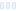# Simply Go Raw

## Nature's Eats

Main info:

Simply Go Raw
Nature's Eats
1 bag
210 Calories
8 g
19 g
7.0 g

4 g
0 mg
1.5 g
0 mg
2 g
0 g

Nutrition Facts
For a Serving Size of (g)
How many calories are in Simply Go Raw? Amount of calories in Simply Go Raw: Calories Calories from Fat (%)
% Daily Value *
How much fat is in Simply Go Raw? Amount of fat in Simply Go Raw: Total Fat
How much saturated fat is in Simply Go Raw? Amount of saturated fat in Simply Go Raw: Saturated fat
How much sodium is in Simply Go Raw? Amount of sodium in Simply Go Raw: Sodium
How much potassium is in Simply Go Raw? Amount of potassium in Simply Go Raw: Potassium
How many carbs are in Simply Go Raw? Amount of carbs in Simply Go Raw: Carbohydrates
How many net carbs are in Simply Go Raw? Amount of net carbs in Simply Go Raw: Net carbs
How much sugar is in Simply Go Raw? Amount of sugar in Simply Go Raw: Sugar
How much fiber is in Simply Go Raw? Amount of fiber in Simply Go Raw: Fiber
How much protein is in Simply Go Raw? Amount of protein in Simply Go Raw: Protein
Vitamins and minerals
How much Calcium is in Simply Go Raw? Amount of Calcium in Simply Go Raw: Calcium
How much Iron is in Simply Go Raw? Amount of Iron in Simply Go Raw: Iron
Fatty acids
Amino acids
* The Percent Daily Values are based on a 2,000 calorie diet, so your values may change depending on your calorie needs.
Percent calories from...Loading similar foods...
Note: Any items purchased after clicking our Amazon buttons will give us a little referral bonus. If you do click them, thank you!Be cool

- Zen orangeI never skip arm day

- Buff broccoli